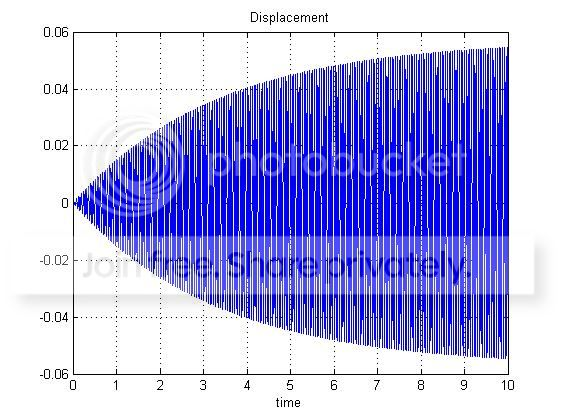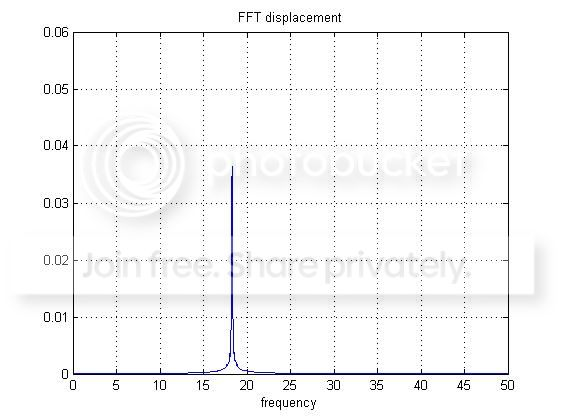# system response: time vs frequency. Why do I get different magnitudes?

I am simulating a linear system described by the following equation

$m \ddot{x} + c \dot{x} + k x = A \sin(\omega t)$

Fs = 1000;      % sampling frequency
tspan = 0:1/Fs:10;
m = 25;
c = 15;
k = 330000;
A = 100;           % forcing amplitude
omega = 114.89;    % forcing frequency

% State space function
odefun = @(t,u)[u(2); 1/m*(-k*u(1)-c*u(2)+A*sin(omega*t))];

[time,u] = ode45(odefun,tspan,[0 0]);

spost = u(:,1);    % displacement
vel = u(:,2);      % velocity


Then I compute the FFT of the displacement:

L = length(spost);
NFFT = 2^nextpow2(L);
SPOST = fft(spost,NFFT)/L;
f = Fs/2*linspace(0,1,NFFT/2+1);


And this is what I get:As you can see the maximum value of the displacement is different in the 2 cases, but this does not look right to me. In fact, if I run a structural analysis in the time or in the frequency domain I would expect to obtain the same maximum displacement (since the excitation frequency is equal to the resonance frequency).

How can this be explained?

EDIT

I forgot to say I tried also increasing the simulation time, but still the 2 values do not match

## 2 Answers

What you are trying to do is to compare the amplitude of your signal with an amplitude of FFT peak, expecting it is the same. The thing is that amplitude of your signal is varying and FFT is taking the "average" over whole duration.

In such case you should use time-frequency representation of your signal, such us spectrogram. It will allow you to observe how amplitude of particular frequency is changing over time. Since you work in MATLAB you can try following code:

win = hamming(256)
noverlap = 0
nfft = 512
X = abs(spectrogram(spost, win, noverlap, nfft))
% Scale by window and multiply by 2 to restore energy from negative freqs
X = 2*X/sum(win)
surf(X)


It will produce something like:The maximum value of displacement is around $0.053$. Results can be tweaked even further by tweaking even more and using other methods.

• That makes sense. And since the Fourier transform is a sort of average over the whole duration, does it mean that the longer the duration, the better the transformed signal? I mean, is the energy content of the signal represented more faithfully if the duration is longer? – Rhei Jan 5 '15 at 14:23
• If by " the longer the duration [...] the energy content of the signal represented more faithfully" you mean: if large amount of samples are at steady state (your target amplitude) the estimate will be dominated with target amplitude, then yes. But it is not a way to go. Exactly for such purposes we have time-frequency analysis. – jojek Jan 5 '15 at 14:39
• What is time-frequency analysis exactly? – Rhei Jan 5 '15 at 15:44
• For example: Short-Time Fourier Transform, Wavelet Transform, Wigner Transform, Short-Time Chirp Fourier Transform, etc. Shortly speaking - anything that allows you to see when and which frequencies are present. Although this question was answered already. – jojek Jan 5 '15 at 15:49
• Thank you, I will have a look to the other Q/A to have a better understanding of the method – Rhei Jan 5 '15 at 15:51

Just to supplement what jojek has explained, there are 2 reasons why your amplitude won't match. First is the envelope/amplitude of you signal. It starts at 0 and then ramps up to 0.058. It appears to follow a $1-e^{-\alpha t}$ behaviour. Since this function is essentially multiplied by your sinusoid in the time domain - that implies a convolution in the frequency domain - this convolution will essentially spread out the peak response that you are expecting i.e. some energy will appear in neighbouring frequency bins. You can reduce this effect by taking the FFT of a longer output sequence, and/or start the FFT from time $t=10$ - this essentially helps reduce the effect of the transient start up.

The second effect may be due to spectral leakage. The FFT only evaluates the response at discrete frequency points. If the frequency of time domain signal does not lie exactly on one of these FFT bins, then you get energy leakage into nearby FFT bins. Again one way to help mitigate this factor is to take the FFT of a longer sequence. A larger FFT means the frequency bins are more closely spaced together.

As you can see from your frequency plot you do not have a sharp peak - there are sidelobes present - which is why you aren't getting the amplitude you are expecting. Some of that energy is leaking into adjacent frequency bins, due to the discrete nature of the FFT frequency bins.

One alternative to help improve the amplitude measurement of the FFT is to use a Flattop window on the data, and take a larger FFT - but use more actual data (don't just zero pad). There is a flattop window available directly in Matlab, or you should be able to find some formulas available online.